Sampling Theorem

When the sampling interval is Δt seconds (sampling is made every Δt seconds), the sampling frequency, fs, is 1/Δt (1/Δt points are sampled a second.) Sampling theory indicates the relationship between a temporally continuous signal and the least possible sampling rate with which the original information can be maintained. It prescribes that the sampling frequency must be at least two times the highest harmonic component contained in the signal. If the sampling frequency is lower than two times the signal frequency, aliasing (aliasing noise) occurs.

Sound Intensity (SI) or Acoustic Intensity (AI)

The sound intensity is the energy of sound which passes a unit cross-section area, including the sound field point, within a given unit of time. It is defined as a vector quantity which is the time average of the product of the sound pressure at point p (t) and the particle velocity, ur (t), into direction r. If the sound intensity into direction r is Ir, the following expression is given.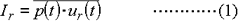In a steady medium with a density of ρ, the relationship between p(t) and ur(t) is represented by expression (2).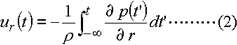However, it is very difficult to measure the particle velocity directly and correctly. To solve this problem, a method to approximate the particle velocity from the difference between sound pressures of two points was considered. This method is the SI measurement method based on two microphones. Using sound pressures p1(t) and p2(t) of the two microphones which are separated by ⊿r into direction r, p (t) and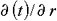can be obtained as an approximate value: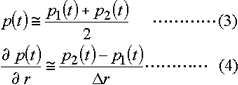When substituting expression (4) into expression (2), the particle velocity into direction r, ur(t), can be represented by expression (5).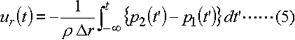Then, the sound intensity, Ir, is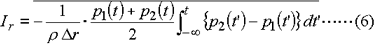This expression calculates Ir directly in the time domain and is referred to as the direct integral method.

In addition, in many cases expression (7) is used to obtain Ir into direction r at any desired frequency band from f1 to f2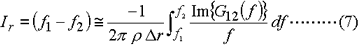where Im{G12(f)} is the imaginary part of the (one-side) cross spectrum of p1(t) and p2(t). If you obtain the cross spectrum between sound pressure signals at two close points using a 2-channel FFT analyzer and then calculate the above expression using the imaginary part, you can obtain the frequency band Ir for any desired frequency band. This approach is referred to as the cross spectrum method.  Measurement errors of the SI measurement method include the limited differential error by limited ⊿r, the sensitivity between two microphones, and the error caused by mismatched phase. Various considerations are made on this correction method.

The following are sample applications using the SI measurement method.

(1) Measuring power level of sound source

The sound intensity represents the amount of sound energy passing in a unit area in a unit time. The total power, P, emitted from the sound source is given by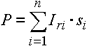(Iri is the sound intensity vertical to plane si, and si is the i-th area.)

The above expression allows you to calculate the sound power based on sound intensity measurement at a split plane, on a hemisphere centering on the sound source and into the direction which is vertical to the surface of the hemisphere.

(2) Sound shield measurement

Through measurement of the transmission power for each section based on SI, you can make quantitative measurement of the sound shielding characteristics of walls consisting of multiple sections and the sound leakage from gaps. Therefore, the SI method is effective for sound shield measurement on site.

(3) Sound field analysis

The SI value is a vector quantity (a quantity with a magnitude and direction). Therefore, by displaying the propagating direction and the magnitude of the sound in 2D or 3D form, you can visualize and capture the flow of sound energy.

Time-Axis Differential/Integral

Differential

For calculation of the first and second order differential values, the 5th order Lagrange's interpolation formula is used. The data for a given point is obtained based on the value of the five points before and after the target point, including that point.

f0, f1, f2, f3, f4, .... are sample data.

First order differential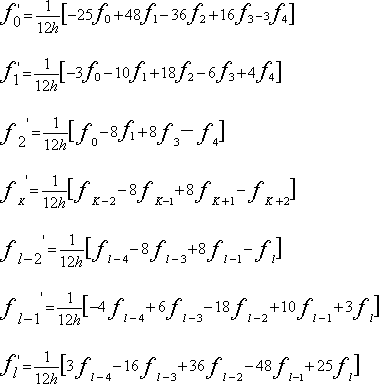Second order differential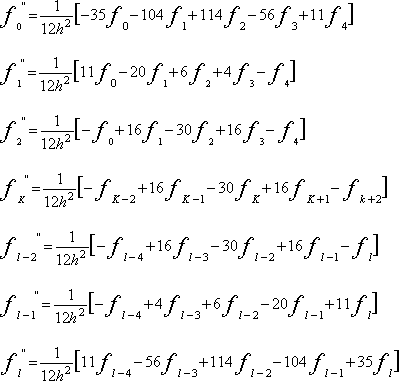Integral

For calculation of the single and double integral values, the trapezoidal formula is used.

Expressions for single integral value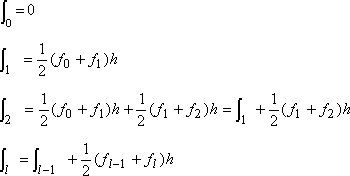Expressions for double integral value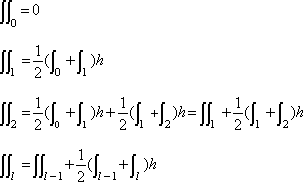Time-Axis Waveform

The instantaneous waveform of the signal input from the input connector of the panel is displayed. The waveform for one frame is displayed. In this case, the X axis is assigned time (seconds) with the starting point of the frame set to 0 and the Y-axis the instantaneous value. The full scale of the X axis is set in conjunction with the frequency range setting.

Time Waveform Statistical Calculation

(1) Mean value (MEAN)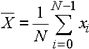(2) RMS value (RMS)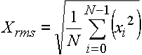(3) Standard deviation (S.D.)

The 2nd order moment around the mean value is referred to as the dispersion, and the square root of the dispersion is referred to as the standard deviation. As for a signal excluding the DC component, the RMS value and standard deviation are equal.  The S.D. is obtained by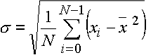The relationship of expressions (1), (2), and (3) is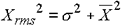(4) Skewness (SKEWNESS)

Skewness denotes the 3rd order moment around the mean value normalized by σ3.  It is used as an index for asymmetry around the mean value. Skewness is obtained by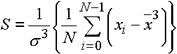(5) Kurtosis (KURTOSIS)

Kurtosis denotes the 4th order moment around the mean value normalized by σ4.  It is used as an index for sharpness of waveform. Kurtosis is obtained by the following formula. Since the value of kurtosis in the time signal of a normal (Gaussian) distribution is 3, the value of kurtosis can be taken as the following equation minus 4.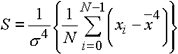(6) Crest factor (CRESTFACTOR)

Obtained by dividing the maximum value by the RMS value.

Trigger Function

The trigger function is a function which starts sampling based on a certain point of an input signal or input of an external signal.

There are two types of trigger modes:  internal trigger mode and external trigger mode.  In the internal trigger mode, the input signal is used as a sampling start timing signal or trigger signal, and sampling starts when the input signal reaches a specified voltage.  In the external trigger mode, an external signal is input as a sampling start timing signal, and sampling starts when the external trigger signal is input.

This function makes it possible to efficiently sample and analyze a desired waveform portion. When averaging a time waveform, the trigger function is used for waveform synchronization.

Trigger polarity

......

Trigger is activated in three different cases: when the signal rises and reaches the specified voltage (+), when it falls and reaches the specified voltage (-), or in both cases.

Trigger position

......

Specifies the number of points before or after the trigger point, at which sampling start. The trigger mode in which sampling starts before the trigger point is called pre-trigger, and the trigger mode in which sampling starts after the trigger point is called post-trigger.

Trigger level

......

Specifies the voltage level at which the trigger is activated.

Trigger type

......

Single trigger, repeat trigger, and one-shot trigger

 Single trigger When trigger is activated, one frame is sampled and then capturing stopped. Repeat trigger Each time trigger is activated, one frame is sampled. Trigger pulses appearing during sampling are ignored. One-shot trigger Once trigger is activated, the trigger-free condition (in which trigger is not activated) results.